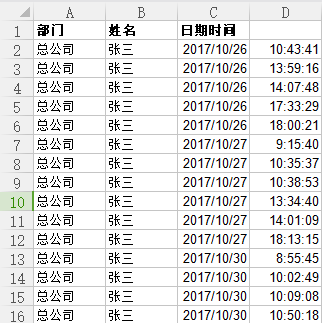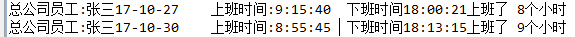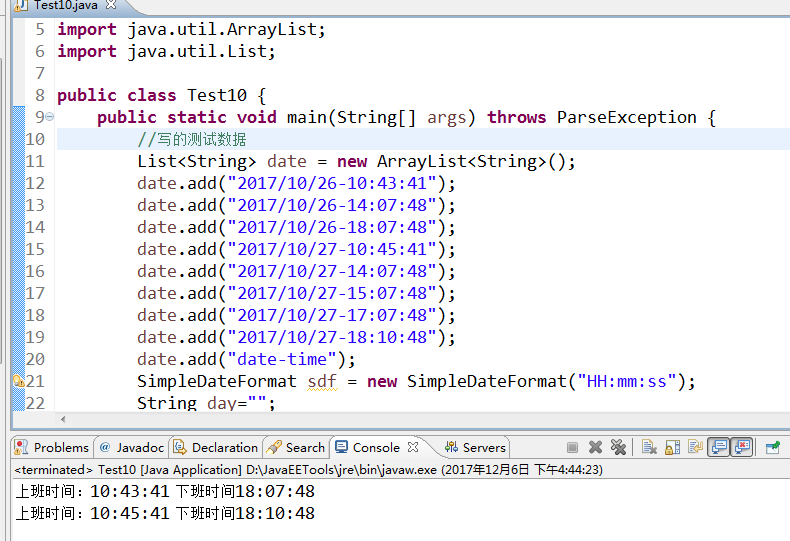`````` import java.io.File;
import java.io.IOException;
import java.text.DateFormat;
import java.text.ParseException;
import java.text.SimpleDateFormat;
import java.util.ArrayList;
import java.util.Date;
import java.util.List;

import jxl.Cell;
import jxl.Sheet;
import jxl.Workbook;

public class daochudaoexcel {
public static void main(String[] args) throws BiffException, IOException, ParseException {
String fileName = "D://Io//jisuan.xls"; // Excel文件所在路径
File file = new File(fileName); // 创建文件对象
Workbook wb = Workbook.getWorkbook(file); // 从文件流中获取Excel工作区对象（WorkBook）
Sheet sheet = wb.getSheet(0); // 从工作区中取得页（Sheet）

int n =0;
int j =0;
int f = 0;

Date max = null;
String   Stringmax = null;
String Stringmin = null;
String zgs = "总公司";

DateFormat df = new SimpleDateFormat("HH:mm:ss");
//规定早上最晚打卡时间
Date time = df.parse("10:00:00");

while(n<sheet.getRows()){
//如果是总公司
if(sheet.getCell(0,n).getContents().equals(zgs)){
//如果姓名相等
if(sheet.getCell(1,n).getContents().equals(sheet.getCell(1,n+1).getContents())){
//如果日期相等
if(sheet.getCell(2,n).getContents().equals(sheet.getCell(2,n+1).getContents())){
//如果第n个时间小于n+1，那么将max等于n+1
if(df.parse(sheet.getCell(3,n).getContents()).getTime()<df.parse(sheet.getCell(3,n+1).getContents()).getTime() ){
max=df.parse(sheet.getCell(3,n+1).getContents());
//将max转成String
Stringmax = sheet.getCell(3,n+1).getContents();
}
}else {
//如果日期不相等那么下个日期的第一个打卡时间 就是min
Date min= df.parse(sheet.getCell(3,n+1).getContents());
//将min转为String类型
Stringmin = sheet.getCell(3,n+1).getContents();
//如果最早打卡时间大于规定早上最晚打卡时间 那么则判定为迟到
if(min.getTime()>time.getTime()){
System.err.print("迟到");
//如果上班时间大于小于八个小时 则说明早退
}else if((max.getTime()-min.getTime())/3600000<8){
System.err.print("早退");
}
//输出员工上班下班时间 和上班多少小时
System.out.println(sheet.getCell(0,n).getContents()+"员工:" +sheet.getCell(1,n).getContents()+sheet.getCell(2,n+1).getContents() + "    "+ "上班时间:" + Stringmin + "  " + "下班时间" + Stringmax + "上班了" + "  " +(max.getTime()-min.getTime())/3600000 + "个小时" );
}
}
}
n++;
}
}
}
``````4个回答

``````    //换了一个逻辑，由于打卡时间是从小到大排列的，那么只需定位一个人同部门 ，同一天的最早和最晚打卡时间即可，参照如下
public static void main(String[] args) throws BiffException, IOException, ParseException {
String fileName = "D://Io//jisuan.xls"; // Excel文件所在路径
File file = new File(fileName); // 创建文件对象
Workbook wb = Workbook.getWorkbook(file); // 从文件流中获取Excel工作区对象（WorkBook）
Sheet sheet = wb.getSheet(0); // 从工作区中取得页（Sheet）

int n =1;//数据行起始值

DateFormat df = new SimpleDateFormat("HH:mm:ss");
//规定早上最晚打卡时间
Date time = df.parse("10:00:00");
//记录一个周期（同一个地区、同一个人、同一天的上下班）数据
String firm="";//公司
String name="";//人员
String date="";//日期
String time_start="";//最早打卡时间
String time_end="";//最迟打卡时间
int hours=0;//上班时间
boolean isBegin=true;
while(n<sheet.getRows()){
//新起始行,记录最早打卡数据
if(isBegin){
firm=sheet.getCell(0,n).getContents();
name=sheet.getCell(1,n).getContents();
date=sheet.getCell(2,n).getContents();
time_start=sheet.getCell(3,n).getContents();
isBegin=false;
}
//找结束行，即最晚打卡数据
else{
String this0=sheet.getCell(0,n).getContents();
String this1=sheet.getCell(1,n).getContents();
String this2=sheet.getCell(2,n).getContents();
//判断和上一行是相同性质行
if(this0.equals(firm) && this1.equals(name) && this2.equals(date)){
//判断是最后一行
if(!sheet.getCell(0,n+1).getContents().equals(this0) || !sheet.getCell(1,n+1).getContents().equals(this1) && !sheet.getCell(2,n+1).getContents().equals(this2)){
time_end=sheet.getCell(3,n).getContents();
hours=(df.parse(time_end).getTime()-df.parse(time_start).getTime())/3600000;
//判断是否迟到
if(df.parse(time_start).getTime()>time.getTime()){
System.err.print("迟到");
}
//判断是否早退
else if(hours<8){
System.err.print("早退");
}
//输出员工上班下班时间 和上班多少小时
System.out.println(firm+"员工:" +name+date+ "上班时间:" + time_start + "  " + "下班时间" + time_end + "上班了" + "  " +hours.toString()+ "个小时" );

//当前数据结束，进行下一次统计
isBegin=true;
}
}
}
n++;
}

}
``````m11418166 回复张大教主: 你这个代码我实在没弄明白 但是按照你的思路 前三条要是一样的话 说明就是一天 自己写了一个 。。m11418166 一行的 永远为false 进不去啊m11418166 很强 你这个思路很不错 要我写都写不出来 但是你写的if(!sheet.getCell(0,n+1).getContents().equals(this0) || !sheet.getCell(1,n+1).getContents().equals(this1) && !sheet.getCell(2,n+1).getContents().equals(this2)){m11418166 回复我是一只小小小小小鸟: 对应该是我这个逻辑有问题 因为我这个遍历 下班时间的时候肯定会让n增加 然后我的打印都是在else中的所以代码到了第一次else的时候n已经是5了m11418166 这个表是按照打卡时间顺序排列的，所以第二天的第一个打卡时间一定是上班时间 最后一个打卡时间肯定是下班时间。但是你不知道这个人打了几次卡啊，所以你不知道第几个是下班时间，你那个最大时间和最小时间什么意思没有太明白``````public static void main(String[] args) throws ParseException {
//写的测试数据
List<String> date = new ArrayList<String>();
SimpleDateFormat sdf = new SimpleDateFormat("HH:mm:ss");
String day="";
String start = null,end = null;
for (int i = 0; i < date.size(); i++) {
String[] time = date.get(i).split("-");
//判断是否到了下一天
if (!day.equals(time)) {
if (start!=null&&end!=null) {
System.out.println("上班时间："+start+"\t下班时间"+end);
}
//把每天第一条数据当做开始和结束时间
day = time;
start = time;
end = time;
}
//只对结束时间做更新
end = time;
}
}
``````m11418166 强 你的方法好简单啊 比我那个好用多了 我怎么写不出来这种代码啊

`````` package com.haxk.fangfa;

import java.io.File;
import java.io.IOException;
import java.text.ParseException;
import java.text.SimpleDateFormat;
import java.util.ArrayList;
import java.util.Date;
import java.util.List;

import jxl.Sheet;
import jxl.Workbook;
import jxl.write.Label;
import jxl.write.WritableSheet;
import jxl.write.WritableWorkbook;

public class zijixiedefangfa {
public static void main(String[] args) throws BiffException, IOException, ParseException {
String fileName = "D://Io//kaoqin.xls"; // Excel文件所在路径
File file = new File(fileName); // 创建文件对象
Workbook wb = Workbook.getWorkbook(file); // 从文件流中获取Excel工作区对象（WorkBook）
Sheet sheet = wb.getSheet(0); // 从工作区中取得页（Sheet）

int n =0;
Date min = null;

String firm = ""; //定义公司
String name = ""; //定义姓名
String day = ""; //定义日期
String time_start = ""; //定义最早时间
String time_end = "";  //定义最晚时间

List shuju = new ArrayList();   //定义总的传入集合
List bumen = new ArrayList();  //部门
List xingming = new ArrayList();   //姓名
List date = new ArrayList();   //日期
List earlyTime = new ArrayList();  //上班时间
List endTime = new ArrayList<Date>();    //下班时间
List workTime = new ArrayList();  //上班时间
List chidao  = new ArrayList();  //迟到
List zaotui  = new ArrayList();   //早退

//最晚上班时间
SimpleDateFormat df = new SimpleDateFormat("HH:mm:ss");
Date biaozhun  = df.parse("10:00:00");

while(n<sheet.getRows()){
//这一天的最早上班时间
firm = sheet.getCell(0,shuju.size()).getContents();
name = sheet.getCell(1,shuju.size()).getContents();
day = sheet.getCell(2,shuju.size()).getContents();
time_start = sheet.getCell(3,shuju.size()).getContents();

//判断是否迟到
if(df.parse(sheet.getCell(3,shuju.size()).getContents()).getTime()>biaozhun.getTime()){
}else{
}

while(n<sheet.getRows()){
//如果前三条数据一样的话 说明是同一天
if(sheet.getCell(0,n).getContents().equals(firm) && sheet.getCell(1,n).getContents().equals(name) && sheet.getCell(2,n).getContents().equals(day) ){
}else{
break;
}
n++;
}
//当前三条数据不一样时 退出当前while循环 数组长度减1的下标就是下班时间
}

for (int i = 0; i < earlyTime.size(); i++) {
try {
//判断是否早退
if((df.parse((String) endTime.get(i)).getTime()-df.parse((String) earlyTime.get(i)).getTime())/3600000>=8){
}else{
}
} catch (ParseException e) {
e.printStackTrace();
}
}
//关闭读取流
wb.close();

try {
//打开写入流
WritableWorkbook book = Workbook.createWorkbook(new File("D://Io//stu.xls"));
// 生成名为“sheet1”的工作表，参数0表示这是第一页
WritableSheet kaoqin = book.createSheet("kaoqin", 0);

//写入部门
for (int i = 0; i < bumen.size(); i++) {
Label label = new Label(0, i,  (String) bumen.get(i));
// 将定义好的单元格添加到工作表中
}

//写入姓名
for (int i = 0; i < xingming.size(); i++) {
Label label = new Label(1, i,  (String) xingming.get(i));
}

//写入日期
for (int i = 0; i < date.size(); i++) {
Label label = new Label(2, i,  (String) date.get(i));
}

//写入上班时间
for (int i = 0; i < earlyTime.size(); i++) {
Label label = new Label(3, i,  (String) earlyTime.get(i));
}

//写入下班时间
for (int i = 0; i < endTime.size(); i++) {
Label label = new Label(4, i,  (String) endTime.get(i));
}

//写入总共上班时间
for (int i = 0; i < workTime.size(); i++) {
Label label = new Label(5, i,   (String) workTime.get(i)) ;
}

//加入迟到

for (int i = 0; i < chidao.size(); i++) {
Label label = new Label(6, i,   (String) chidao.get(i)) ;
}

//写入早退
for (int i = 0; i < zaotui.size(); i++) {
Label label = new Label(7, i,   (String) zaotui.get(i)) ;
}

book.write();    //执行写入操作
book.close();     //关闭流

}catch (Exception e) {
System.out.println(e);

}
}
}
``````java考勤系统算法问题

java tcp实现模拟考勤打卡代码怎么写

[MBean{sign_in_start_time='2018-10-26 08:00:00', sign_in_end_time='2018-10-26 10:00:00', sign_out_start_time='2018-10-26 11:00:00', sign_out_end_time='2018-10-26 13:00:00'}, MBean{ sign_in_start_time='2018-10-26 14:30:00', sign_in_end_time='2018-10-26 15:30:00', sign_out_start_time='2018-10-26 19:42:00', sign_out_end_time='2018-10-26 21:42:00'}] 这个集合里面有两个对象元素，里面是当天需要打卡考勤的时间段，就是说这个人一天要打4次卡，在界面上需要显示两个字段，第一个班次需要显示的效果： 上班时间：2018-10-26 08:00:00-2018-10-26 10:00:00 下班时间：2018-10-26 11:00:00-2018-10-26 13:00:00 假如第一个班次的上班时间过了后，就应该显示： 下班时间：2018-10-26 11:00:00-2018-10-26 13:00:00 上班时间：2018-10-26 14:30:00-2018-10-26 15:30:00 假如第一个班次的下班时间过了后，就应该显示： 上班时间：2018-10-26 14:30:00-2018-10-26 15:30:00 下班时间：2018-10-26 19:42:00-2018-10-26 21:42:00 以此类推，不管这个人有几个班次都可以这样子实现。 请问这种逻辑应该怎么实现，我想的都不完美。求大佬帮帮忙

1. ```FOR DELETE,Update AS 1. delete from onoffdutydata where onoffdutyid in ( select a.onoffdutyid From onoffdutd in ( select a.onoffdutyid From onoffdutydata a inner join employeemsg b on a.employeeid=b.employeeid and a.checkdate<b.ondutytime) 1. delete from employeesalary where salaryid in ( select a.salaryid From employeesalary a inner join employeemsg b on a.employeeid=b.employeeid and a.Yearmonth>convert(nvarchar(7),outdutytime,21)) 1. delete from employeesalary where salaryid in ( select a.salaryid From employeesalary a inner join employeemsg b on a.employeeid=b.employeeid and a.Yearmonth<convert(nvarchar(7),ondutytime,21)) 1. delete from originalcheckdata where originaldataid in (select a.originaldataid from originalcheckdata a inner join originalcheckdata b on a.employeeid=b.employeeid and a.occurtime=b.occurtime and a.originaldataid>b.originaldataid) GO SET QUOTED_IDENTIFIER OFF GO SET ANSI_NULLS ON GO ``` ![图片说明](https://img-ask.csdn.net/upload/201912/20/1576841900_935726.png)
sql 组语句 关于考勤的计算

web考勤系统如何给员工电脑加认证

java操作中控考勤机 获取数据

JAVA Web员工考勤统计

php 开发企业考勤系统

Java学习的正确打开方式

linux系列之常用运维命令整理笔录

Python十大装B语法
Python 是一种代表简单思想的语言，其语法相对简单，很容易上手。不过，如果就此小视 Python 语法的精妙和深邃，那就大错特错了。本文精心筛选了最能展现 Python 语法之精妙的十个知识点，并附上详细的实例代码。如能在实战中融会贯通、灵活使用，必将使代码更为精炼、高效，同时也会极大提升代码B格，使之看上去更老练，读起来更优雅。 1. for - else 什么？不是 if 和 else 才

2019年11月中国大陆编程语言排行榜
2019年11月2日，我统计了某招聘网站，获得有效程序员招聘数据9万条。针对招聘信息，提取编程语言关键字，并统计如下： 编程语言比例 rank pl_ percentage 1 java 33.62% 2 c/c++ 16.42% 3 c_sharp 12.82% 4 javascript 12.31% 5 python 7.93% 6 go 7.25% 7

SQL-小白最佳入门sql查询一

【图解经典算法题】如何用一行代码解决约瑟夫环问题

“狗屁不通文章生成器”登顶GitHub热榜，分分钟写出万字形式主义大作

IT界知名的程序员曾说：对于那些月薪三万以下，自称IT工程师的码农们，其实我们从来没有把他们归为我们IT工程师的队伍。他们虽然总是以IT工程师自居，但只是他们一厢情愿罢了。 此话一出，不知激起了多少(码农)程序员的愤怒，却又无可奈何，于是码农问程序员。 码农：你知道get和post请求到底有什么区别？ 程序员：你看这篇就知道了。 码农：你月薪三万了？ 程序员：嗯。 码农：你是怎么做到的? 程序员：
《程序人生》系列-这个程序员只用了20行代码就拿了冠军

11月8日，由中国信息通信研究院、中国通信标准化协会、中国互联网协会、可信区块链推进计划联合主办，科技行者协办的2019可信区块链峰会将在北京悠唐皇冠假日酒店开幕。 　　区块链技术被认为是继蒸汽机、电力、互联网之后，下一代颠覆性的核心技术。如果说蒸汽机释放了人类的生产力，电力解决了人类基本的生活需求，互联网彻底改变了信息传递的方式，区块链作为构造信任的技术有重要的价值。 　　1

【技巧总结】位运算装逼指南

8年经验面试官详解 Java 面试秘诀
作者 | 胡书敏 责编 | 刘静 出品 | CSDN（ID：CSDNnews） 本人目前在一家知名外企担任架构师，而且最近八年来，在多家外企和互联网公司担任Java技术面试官，前后累计面试了有两三百位候选人。在本文里，就将结合本人的面试经验，针对Java初学者、Java初级开发和Java开发，给出若干准备简历和准备面试的建议。   Java程序员准备和投递简历的实

1.两种思维方式在求职面试中，经常会考察这种问题：北京有多少量特斯拉汽车？ 某胡同口的煎饼摊一年能卖出多少个煎饼？ 深圳有多少个产品经理？ 一辆公交车里能装下多少个乒乓球？ 一